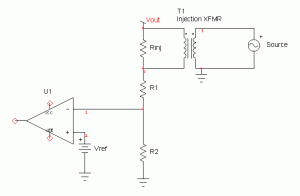top of page
Search

# FAQ #18: What injection resistor value should I use? Is it okay to use a little larger resistor like

What injection resistor value should I use? Is it okay to use a little larger resistor like 30 or 50 ohm instead of 5 ohm (like many app notes recommend)?There are a wide variety of opinions, ranging from “it should match the source impedance of 50 Ohms) to “as small as possible”. It may seem arbitrary, and to some extent it is, but there are tradeoffs to consider.

1)  Will this resistor be included in the circuit all the time? If so when no injection transformer is connected, it appears in series with the voltage divider and when the injection transformer is connected it is not. For this reason the value of the resistor should be very small compared with the divider resistor it is connected in series with.

2) The low frequency limit of the injection signal is set by the L/Rinj ratio, where L is the injection transformer inductance. Since we wish XL to be at least 5 times greater than the resistor, the lower the resistor value the lower the -3dB frequency at the low end.

3) Many analyzers cannot go to very small signal levels and so this resistor also forms an attenuator with the source impedance of the oscillator (typically 50 Ohms). The most common bode plot error is that the injected signal is too large.   A lower value injection resistor also helps by providing additional attenuation.Figure 2: Injection transformer with 5 Ohm (dashed) and 50Ohm (solid) terminations showing that the terminating resistor is an integral part of determining the transformer frequency response. (Plot using OMICRON Labs Bode-100).

While there are these tradeoffs to consider, the value we recommend and use ourselves in most applications is 4.99 Ohms.

The low frequency limit is set by the L/Rinj ratio, where L is the injection transformer inductance. Since we wish XL to be at least 5 times greater than the resistor, the lower the resistor value the lower the -3dB frequency at the low end. Also, many analyzers cannot go to very small signal levels (The E5061 and Bode 100 can) and so we also like the attenuation (insertion loss) resulting from the smaller resistor.

A big issue with many Bode plots is that injection signals that are too large. This is one reason why Picotest makes the J2140A Attenuators. In PFC applications we often have to measure down to 1 Hz and so this becomes critical. At 5 Hz, it is somewhat less important. Also note that Picotest injection transformers are CE certified for CAT II 600V so it is safe to measure non-isolated PFC’s using these transformers.

 “Why Network Analyzer Signal Levels Affect Measurement Results”, November 2010 – https://www.picotest.com/blog/